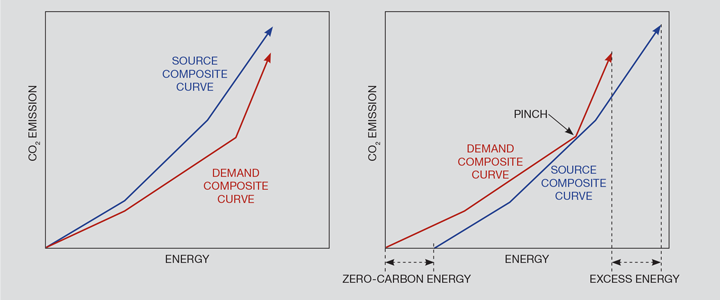# Carbon: Feeling the Pinch

Article by Raymond Tan and Dominic Foo CEng FIChemE

How pinch analysis techniques can be used to optimise decarbonisation techniques

CLIMATE change has emerged as a major environmental issue for the international community. According to a recent report by the Intergovernmental Panel on Climate Change1, net global emissions of CO2 and other greenhouse gases (GHGs) need to be cut to zero by mid-century in order to safely stabilise global warming to about 1.5˚C by 2100. Such reductions can only be achieved by large-scale deployment of decarbonisation techniques, including supply- and demand-side energy efficiency enhancement, fuel switching, increased use of renewables and nuclear energy, and commercialisation of carbon capture, storage and utilisation (CCUS) technologies. Given the potentially difficult choices that need to be made in large-scale decarbonisation programmes, policy makers can benefit from techniques to estimate, visualise and communicate how deep GHG emissions cuts can be achieved.

Within the chemical engineering community, we now have some established tools that can be used for planning the above decarbonisation techniques. This includes the various forms of process integration techniques (including pinch analysis and mathematical optimisation), which were originally developed in the 1970s for minimising energy requirements in industrial plants. The methodology has since been extended to address various problems involving minimisation of resource use and waste generation. One of the more recently-developed branches of process integration is carbon emission pinch analysis (CEPA), which determines optimal allocation of energy in carbon-constrained systems. This article looks at some of the established CEPA techniques.

## CEPA graphical methodology

The original CEPA methodology is based on a graphical pinch analysis technique known as the energy planning pinch diagram (EPPD), which the authors originally proposed for high-level planning of carbon-constrained energy systems.2 The method has since been widely used by researchers to analyse problems in six different continents.3

CEPA methodology deals with the two-fold problem of determining the minimum amount of zero- or low-carbon energy source needed to meet the energy requirements and emissions constraints of a system; and determining the allocation of energy sources in order to satisfy both energy requirements and carbon constraints within the system. Here we assume the methodology applies only to electricity, so that other than the carbon footprint factors, different power sources are practically interchangeable with each other. The main steps of the procedure are:

• Step 1. Define the system boundary and components.
• Step 2. Rank the energy sources in order of ascending carbon intensity.
• Step 3. Plot the energy sources in sequence as vectors on rectangular coordinates, with cumulative power as x-axis and emissions rate as the y-axis. The slope of each segment of this source composite curve corresponds to its carbon intensity.
• Step 4. Rank the energy demands in order of ascending carbon intensity limit.
• Step 5. Plot the energy demands as in Step 3 to generate the demand composite curve. In case the system has a single unified demand for energy, the demand composite curve is just a diagonal line. The horizontal distance of the demand composite curve represents the total energy requirement of the entire system, while its vertical distance represents the total emission limit
• Step 6. Superimpose the two composite curves and note their relative orientation. If the source composite curve is completely below and to the right of the demand composite curve, then the solution is feasible. Otherwise, if the source composite curve crosses the demand composite curve so that part (or even all) of its length is above the latter (which means that the limit has been exceeded), then the initial solution is infeasible, as illustrated in Figure 1a. Such an orientation signifies violation of carbon emissions constraints.
• Step 7. The system’s carbon emissions can be met by introducing zero-carbon energy sources and distributing this new energy source to different energy demands. In general, since the introduction of this new energy source is disruptive to the system, it is necessary to determine the minimum amount needed to meet the emissions limits. This amount can be determined by horizontally shifting the source composite curve to the right, until a feasible geometric orientation as described in Step 6 is reached. The distance of this horizontal shift is the minimum required zero-carbon energy, and is referred to in the CEPA literature as the “target”. Once this orientation is reached, the two composite curves will be at a tangent to each other at the pinch point (see Figure 1b). The latter point serves as a guide for problem decomposition to determine how energy should be allocated. A portion of the source composite curve may protrude to the right of the demand composite curve. The length of this section gives the amount of high-carbon energy sources that need to be eliminated from the system. Figure 1b illustrates the features of an optimal pinch diagram.
• Step 8. Generally, the features determined in Step 7 can then be used to find the proper allocation of energy sources to different energy demands. This final step is trivial in cases where there is only one demand.Figure 1: (a, left) An infeasible EPPD; (b, right) A feasible EPPD with minimum zero-carbon energy and excess energy targets identified

Other than the graphical approach described here, CEPA can also be implemented algebraically with the aid of spreadsheets or using automated targeting models (ATM) in optimisation software. The alternative approaches are mathematically equivalent, but carry advantages and disadvantages. Further details of CEPA methodology are described fully, along with sample applications in different countries, in a forthcoming book.3

## Recent Editions

Catch up on the latest news, views and jobs from The Chemical Engineer. Below are the four latest issues. View a wider selection of the archive from within the Magazine section of this site.